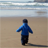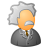# QlikView App Dev

Discussion Board for collaboration related to QlikView App Development.

Announcements
We value your feedback – take our 5-minute QlikView modernization survey
cancel
Showing results for
Did you mean:Creator

## Getting error in expression ')' expected but verified all the braces all are balanced. what could be the reason

Getting error in expression ')' expected but verified all the braces all are balanced. what could be the reason

9 RepliesSpecialist II

Share the expression hereCreator III

If expression is not satisfied it will shows this error.

means it require dimension or expression in ()Partner - Champion III

If you are getting this while running a reload script, recent versions of QlikView will point to the location where a token was (un)expected. Things you can verify

• Function parameter lists: do not specify parameters if the function doesn't accept any
• Function parameter list: did you forget a comma somewhere to separate two parameters?
• \$-sign expansion: do variables and/or expressions expand properly?
• Nested IF() calls: in multiple nested IF() calls, it's easy to lose track of parenthesis balancing
• ...

But the better suggestion is to post your expression for us to analyse.

Best,

PeterCreator
Author

PICK(MATCH(VALUELIST(\$(v_FY_PREM_REV_Concat)),\$(v_FY_PREM_REV_Concat))

,\$(=\$(eRank(Class = {Premium};KPI = {Actual};Year = {"\$(=YEAR(TODAY()))"};[Region Code] = {NA})))

,\$(=\$(eRank(Class = {Premium};KPI = {Actual};Year = {"\$(=YEAR(TODAY())-1)"};[Region Code] = {NA})))

,\$(=\$(eRank(Class = {Premium};KPI = {Actual};Year = {"\$(=YEAR(TODAY())-2)"};[Region Code] = {NA})))

,' '

,''

,\$(=\$(eRank(Class = {Premium};KPI = {Actual};Year = {"\$(=YEAR(TODAY()))"};[Quarter] ={Q1;Q2};[Region Code] = {NA})))

,\$(=\$(eRank(Class = {Premium};KPI = {Actual};Year = {"\$(=YEAR(TODAY())-1)"};[Quarter] ={Q1;Q2};[Region Code] = {NA})))

,\$(=\$(eRank(Class = {Premium};KPI = {Actual};Year = {"\$(=YEAR(TODAY())-2)"};[Quarter] ={Q1;Q2};[Region Code] = {NA})))

)Specialist II

Hi,

in the editor, don't you have a red line ?

may be this a " instead of ' ' :regardsPartner - Champion III

I guess you are unable to figure out where to look in the first place. An approach could be to gradually disable the different complex components of your expression. For example, an edit like this:

PICK(MATCH(VALUELIST(\$(v_FY_PREM_REV_Concat)),\$(v_FY_PREM_REV_Concat)), 1, 2, 3, 4, 5)

would produce garbage. But if it is accepted as an expression, you can be sure that the problem is in the PICK parameter list, and not in the MATCH parameter list.

If it doesn't work with this simplication, you can then try something like:

PICK(MATCH(VALUELIST(\$(v_FY_PREM_REV_Concat)), '1', '2', '3', '4', '5'), 1, 2, 3, 4, 5)

If that works, then you can be sure that the problem is situated somewhere in the expansion of v_FY_PREM_REV_Concat into a list of parameters.

And so on...

Best,

PeterSpecialist III

hello

if i paste the expression in editor, it says "Expression OK"

you should take a look at the values of

v_FY_PREM_REV_Concat,v_FY_PREM_REV_Concat

to check if the syntax error is not contained in one of themCreator
Author

cheked this it is correctmay be while pasting it here in this editor it looked like double quoteCreator
Author

this variable has : vKPI_YTD_Value = 'YTD-17','YTD-16','YTD-15','FY-17','FY-16','FY-15'